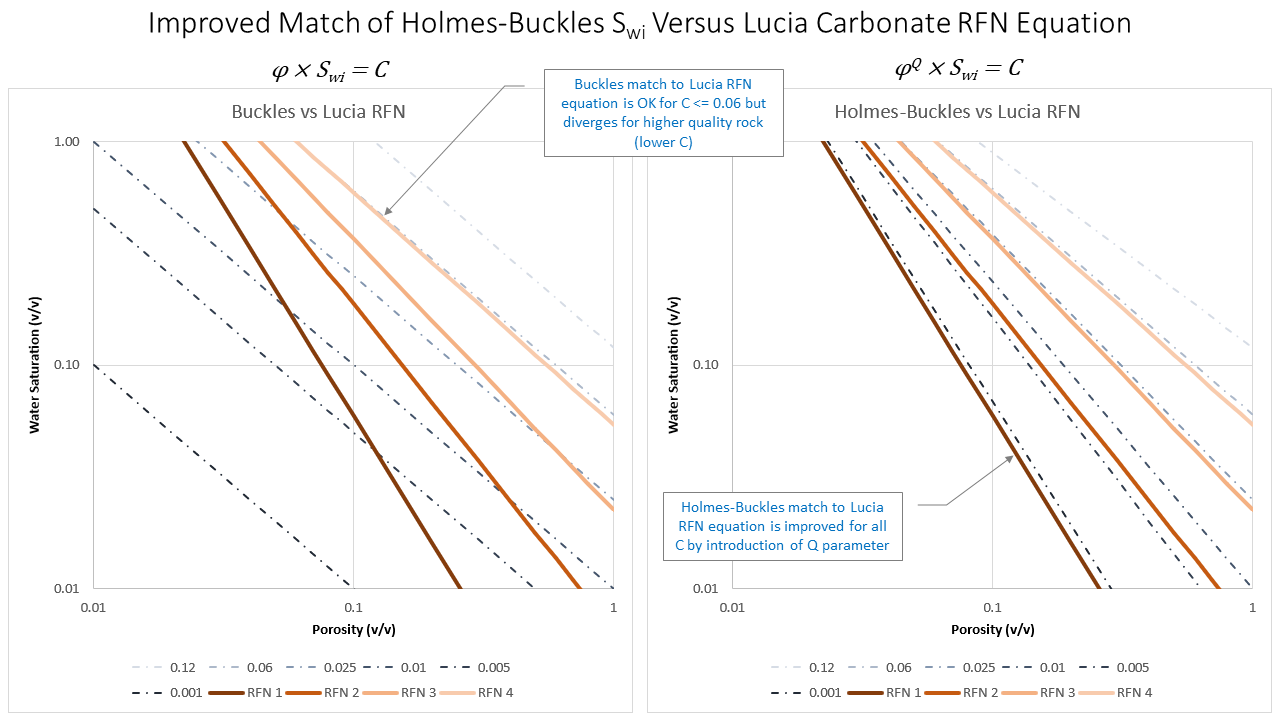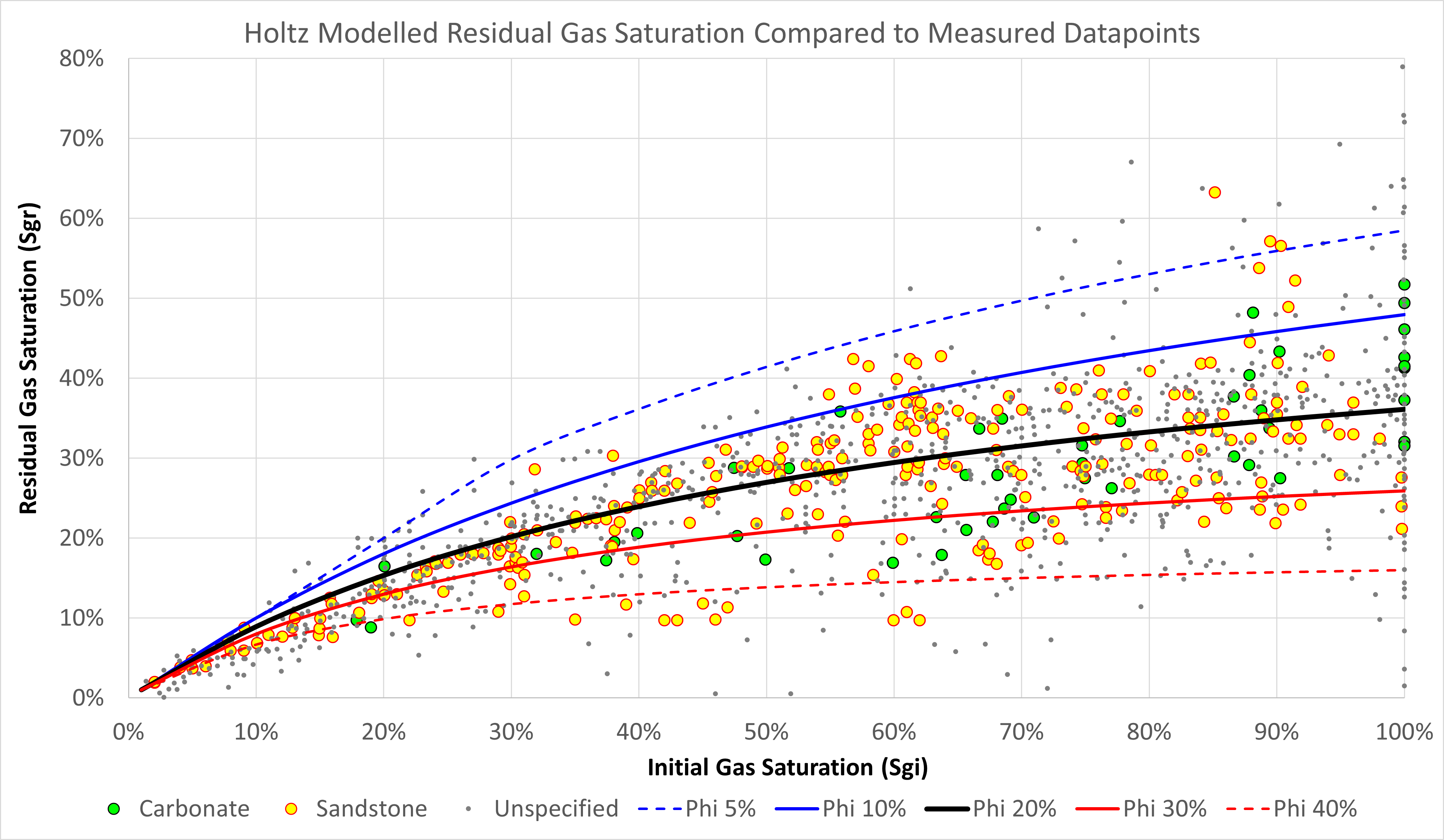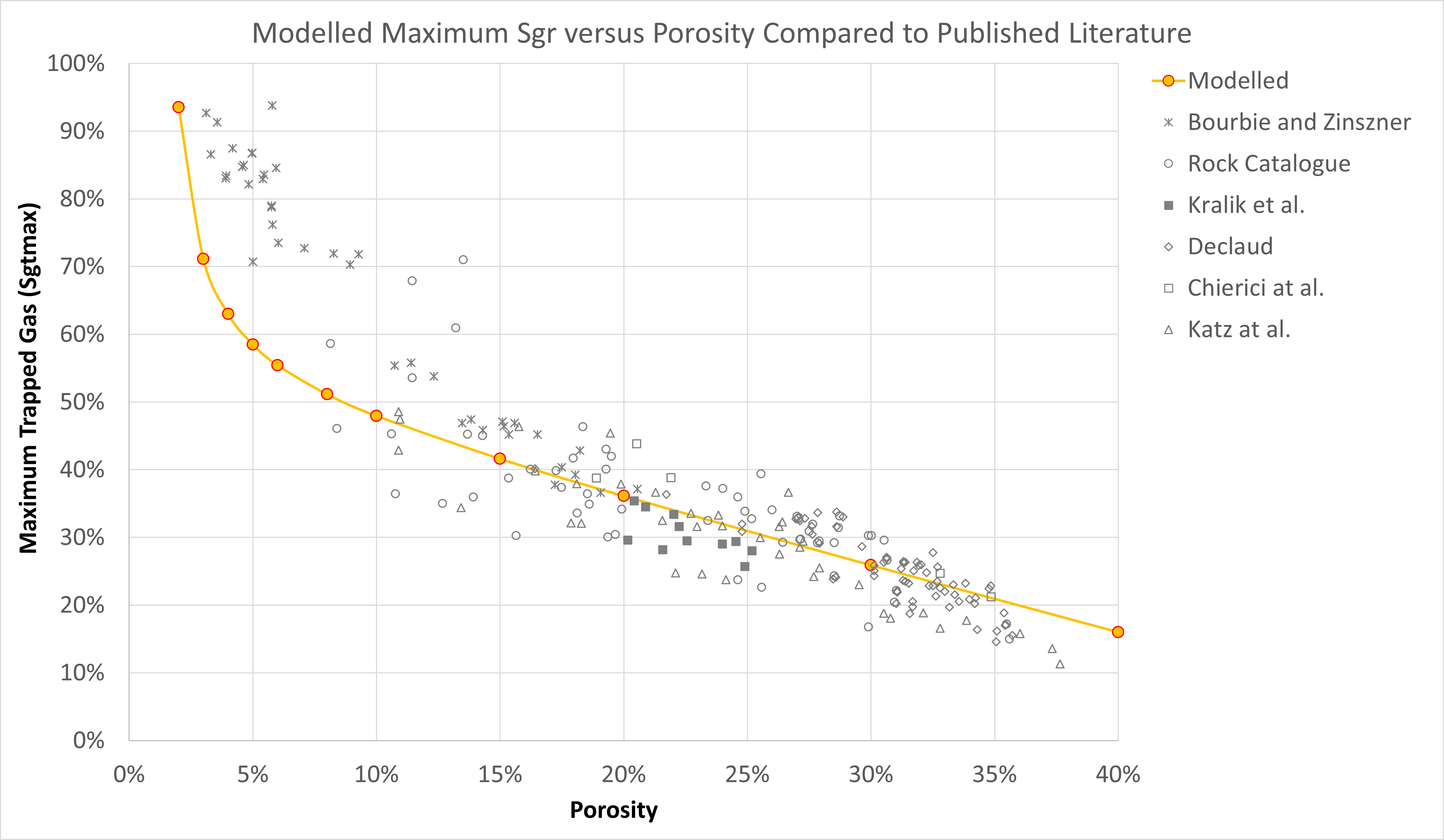# Modelling Saturation Endpoints

For a three-phase system, there are several endpoints that must be considered. These are the irreducible or residual saturations for a fluid where it is not possible to reduce the saturation below this value, and the critical saturations which are the saturation at which a fluid starts to flow.

For our static modelling approach, the objective is to create a framework whereby all reservoir engineering properties can be derived directly, or indirectly, from a rock model that is defined by geology. Therefore variations in the rock properties with respect to the rock character can be defined and our saturation endpoints will adjust such that they are physically consistent with the rock model.

## Irreducible Water Saturation

The irreducible water saturation (Swirr) and the critical water saturation (Swcr) are usually taken as the same value. The irreducible water saturation can be determined using the Holmes-Buckles relationship. The Holmes-Buckles relationship is based on the classical Buckles equation where porosity times irreducible water saturation is a constant. The equation is modified to introduce a porosity exponent.Where:

φ = Rock porosity, fraction
Q = Porosity exponent, dimensionless
Swirr = Irreducible water saturation, fraction
C = Buckles constant, dimensionless

Holmes indicates that the value of Q can vary between 0.8 to 1.3, and that for many reservoirs a value of Q = 1 i.e., the original Buckles formula, is reasonable. A more significant variable in the equation is the constant, or the Buckles number. Holmes notes that ranges for the constant are:

• Sandstones: 0.02 to 0.10
• Inter-granular carbonates: 0.01 to 0.06
• Vuggy carbonates: 0.005 to 0.06

Typically, the higher the constant, the smaller the average pore throat size within the rock matrix. In the absence of reliable data, a guide to the Buckles number can be determined from the rock type:

Buckles Number Sandstone Carbonate
0.12 Very fine grain Chalky
0.06 Fine grain Cryptocrystalline
0.03 Medium grain Inter-crystalline
0.02 Coarse grain Sucrosic
0.01 Conglomerate Fine Vuggy
0.005 Unconsolidated Coarse Vuggy
0.001 Fractured Fractured

The values for C and Q should ideally be determined from petrophysical analysis. A plot of Swirr versus porosity will reveal to most appropriate values to use. In practice, particularly with high permeability carbonates that experience massive drilling fluid losses it can be difficult to reliably ascertain the true Swirr and porosity, in which case a method to calculate a proxy for C from other rock data is required.

The following relationships for sandstones and carbonates have been devised by considering the recommended Buckles numbers for different rock types and comparing these against typical cementation exponents for those rock types. These relationships are designed to capture the expected behaviour of different rock types, but it is cautioned that they have not been extensively, let alone rigorously, tested against large rock datasets.for carbonate m < 1.76for carbonate m ≥ 1.76for sandstone

To determine the parameter Q the default value = 1.0 (as per the original Buckles equation) is used for sandstones. For carbonates, Lucia’s recommended relationship between rock fabric number and water saturation is used to establish a relationship between Q and C. As shown in Figure 1, the introduction of Q into the Holmes-Buckles equation, and use of the following equation to determine Q, allows an excellent match to Lucia’s published relationships.Figure 1: Improvement to irreducible water saturation prediction using Holmes-Buckles equation and comparison to Lucia carbonate trends.

This is perhaps not too surprising as Lucia’s rock fabric number (RFN) equations have a similar form to the Holmes-Buckles equation. It is encouraging that the Holmes-Buckles approach and the Lucia RFN approach do not contradict each other, and it lends support to the assertion that irreducible water saturation can be fundamentally derived from knowledge of the rock porosity and the nature of its pore geometry.

In practice Lucia’s relationships can lead to very high irreducible saturations. The consequence of this is that when creating a static grid using this relationship, the resultant SWCR value can be so high as to render the entire simulation unviable. A set of compromise relationships between the Buckles relationship and the Holmes-Buckles relationship that honours the Lucia relationship is therefore proposed:Using this approach allows C and Q to be determined from the cementation exponent alone, so irreducible water saturation is a function of porosity and cementation exponent which implies that this property is physically dependent on porosity and the pore geometry only. It should be expected that pore throat size has some influence on irreducible water saturation, and that the Buckle’s ‘C’ constant is therefore a function of both cementation exponent and pore throat size. As implemented, this dependency has not been considered. Since our rock model can be used to derive the irreducible water saturation without any other inputs, the rock properties are the only inputs that need to be defined within a static model cell.

## Residual Gas Saturation

For our residual saturations, we are interested in the residual gas saturation (after water invasion) and the residual oil saturation (after both water invasion and gas invasion).

For the residual gas saturation we can use the method proposed by Holtz (2002) which modified the Land equation to produce a relationship that means the residual gas saturation is always equal to or less than the initial gas saturation. The method generates a family of curves depending on the rock quality, with higher porosity rock yielding lower residual gas saturation.

Gas trapping mechanisms are varied, and research suggests that high porosity rock can, under certain circumstances, have high residual or trapped gas as a result of ‘snap-off’ where large pore spaces which are connected by small pore throat sizes are bypassed by lower porosity but larger pore throat pathways. This is a phenomenon that is more likely observed with moldic porosity (separate non-touching vugs) and where the permeability related to the non-touching vugs falls below the average permeability for a given porosity. Therefore, there is preferential flow through the non-vug portion of the matrix.

For our purposes, we are more concerned with modelling a sensible behaviour that is in line with published data sources. The potential for higher residual gas saturation with higher porosity is not considered to simplify the model. Consequently we should expect that there is a relationship between increased residual gas saturation as initial gas saturation increases (as per Land). Similarly, we should expect that as porosity increases (and so does permeability), the pore spaces can be more readily swept by the wetting fluid and thus the residual gas saturation is lower for a given initial saturation.

This is the basis for the Holtz equation given by:Where:

Sgr = Residual gas saturation, fraction
Sgtmax = Maximum trapped gas saturation, fraction
Swirr = Irreducible water saturation, fraction
Sgi = Initial gas saturation, fraction

Holtz suggests a simple relationship between Sgtmax and porosity for use in the residual gas saturation equation:Where:

φ = Rock porosity, fraction

Using these equations gives a series of Land’s curves for residual gas saturation versus initial gas saturation as shown below. The comparison datapoints are taken from a variety of datasets, including both published and unpublished data, and comprise more than 1,200 pairs of values. It can be seen that there is no clear distinction between sandstones and carbonates and that the modelled relationships fall wholly within the bounds of the datapoints.Figure 2: Comparision of modelled residual gas saturation using Holtz equation at different porosities against measured datapoints.

The range of curves generated fits the spread of data observations for different rock types. The endpoints for these lines at 100% initial gas saturation, should also match the measured maximum trapped gas versus porosity. The modelled endpoints are plotted against published data points and exhibit a reasonable fit.Figure 3: Validation that variation of maximum trapped gas saturation with porosity implied by Holtz equation is consistent with published datasets.

The residual gas saturation is a function of porosity and initial gas saturation. The latter is directly related to the irreducible water saturation through a saturation height function, and therefore residual gas saturation is a function of the three properties that define our simple rock model: porosity, cementation exponent and pore size. Therefore, changing our rock model will affect the residual gas saturation in a physically consistent manner.

## Residual Oil Saturation

The residual oil saturation will vary depending upon whether the displacing fluid is water or gas. The wettability of the rock must also be taken into consideration. This gives us two different residual oil saturations:

• Sorw: Residual oil saturation (after water invasion), fraction
• Sorg: Residual oil saturation (after gas invasion), fraction

Let’s consider first Sorw, the residual oil saturation after water invasion. Here the oil and the displacing fluid are immiscible. For a water-wet rock, whereby water adheres to the rock matrix, the residual oil saturation can be considered to be the same as the residual gas saturation. For an oil-wet rock, where the oil-water contact angle > 90 degrees) we might expect the behaviour of the residual oil to be similar to water as the wetting phase in a water-wet rock i.e. Sorw = Swirr.

The situation for the residual oil saturation after gas invasion is different. In this scenario we must consider carefully the miscibility between the gas and oil phases. For a gas-condensate above the dewpoint pressure, the oil and gas phases can be considered miscible. Theoretically, at least, all the oil could therefore be displaced meaning the residual oil saturation is zero. On the other hand, at low pressure and temperature the oil and gas become immiscible and the fluids will behave more like the case of oil being displaced by water. In this case the wettability of the rock again becomes an important consideration.

Al-Nuaimi et al. (2018) published an interesting approach to modelling the change in Sorg in relation to the interfacial tension (IFT) between gas and oil using a Michaelis Menten Kinetics model. As IFT decreases, the residual oil saturation to gas approaches zero.Where:

Sorg,imm = Residual oil saturation to gas under immiscible conditions, fraction
σ = Interfacial tension, dynes/cm
σcrit = Critical interfacial tension = 0.15, dynes/cm

This equation produces a relationship whereby there is a sharp reduction in Sorg below the critical IFT, and a nearly constant Sorg above this point. Measuring σcrit directly is difficult as it requires finding the IFT at which Sorg = Sorg,imm / 2. The recommended approach in the paper is to estimate it using two Sorg measurements. One at immiscible conditions and one at partially miscible conditions. This is not possible with a modelled approach as there are no actual measurements from which to derive the critical interfacial tension. Therefore, for the purposes of our model we will simplify this even further and observe that we are simply looking for a reduction in Sorg under miscible conditions. We set σcrit to a fixed value of 0.15 and from this we can determine the miscible Sorg.

Unlike irreducible water saturation or residual gas saturation, the residual oil saturation is a function of fluid properties only and is independent of any rock properties. Therefore, changing the rock model by altering porosity, cementation exponent or pore size should have no effect on the residual oil saturation. This makes sense as the residual oil saturation is related to miscibility between hydrocarbon phases in the pore volume, and is not related to wettability of the pore space within the rock.### Written by Peter Kirkham

Upstream jack-of-all-trades and master of some.

Updated